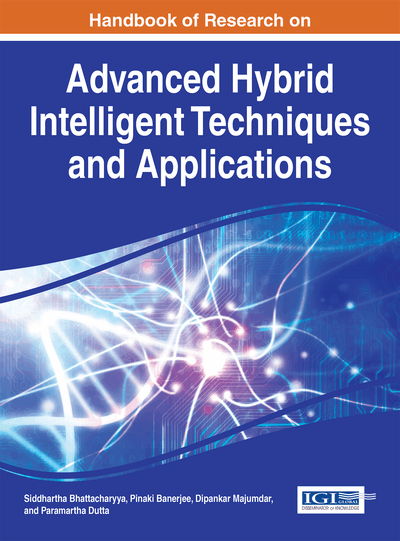# Detailed Analysis of Ultra Low Power Column Compression WALLACE and DADDA Multiplier in Sub-Threshold Regime

Priya Gupta (BITS, India), Anu Gupta (BITS, India) and Abhijit Asati (BITS, India)
DOI: 10.4018/978-1-4666-9474-3.ch004

## Abstract

In this chapter, the design and comparative analysis is done in between the most well-known column compression multipliers by Wallace and Dadda in sub-threshold regime. In order to reduce the hardware which ultimately reduces area, power and overall power delay product, an energy efficient basic modules of the multipliers like AND gates, half adders, full adders and partial product generate units have been analyzed for sub-threshold operation. At the last stage ripple carry adder is used in both multipliers. The performance metrics considered for the analysis of the multipliers are: power, delay and PDP. Simulation studies are carried out for 8x8-bit and 16x16-bit input data width. The proposed circuits show energy efficient results with Spectre simulations for the TSMC 180nm CMOS technology at 0.4V supply voltage. The proposed multipliers so implemented outperform its counterparts exhibiting low power consumption and lesser propagation delay as compared to conventional multipliers.
Chapter Preview
Top

## Introduction

During the last decade, extensive consideration has been given to the use of hybrid intelligence design technique which is suitable to design energy efficient digital modules for very large scale integration (VLSI) implementation. The computing efficiency of this modern technique offers a highly efficient solution to the hybrid intelligence based applications, digital signal processing (DSP) processors and biomedical applications like hearing aids, pacemakers etc.

In order to maintain the rapid increase of energy efficient fidelity applications, emphasis will be on incorporation of low power energy efficient modules in future system design. The design of such modules will have to partially rely on reduced power dissipation in fundamental hybrid intelligence units. Most of the hybrid intelligent applications need to design low power arithmetic circuit towards the development of power-efficient systems such as adders and multipliers. These underscores urges us to design a low power multipliers.

Multiplication is often an essential function in digital systems. Low power multiplication has always been a fundamental requirement of energy efficient processors and systems. In DSP application, multiplications are one of the most utilized arithmetic operations, as part of filters, convolvers, and transform processors. Improving multiplier design directly benefits the low power embedded processors used in consumer and industrial electronic products. In the past five decades, engineering ingenuity has moved multiplication away from the slow add-and-shift techniques to faster, parallel multiplication schemes. In the first large-scale digital systems, multiplication was performed as a series of additions and shifts. The requisite hardware consisted only of a parallel adder and a few registers.

The performance of multiplier was significantly improved with the introduction of Booth’s method proposed by (Andrew D. Booth, 1951). Booth’s method and the modified Booth method do not require a correction of the product when either (or both) of the operands is negative for two’s complement numbers. During the 1950’s, multiplier designs moved away from the slow sequential multiplication to faster simultaneous or parallel multipliers.

The two classes of parallel multipliers were defined by (R. De Mori, 1965). The first class of parallel multiplier uses a rectangular array of identical combinational cells to generate and sum the partial product bits. Multipliers of this class are called iterative array multipliers or, more simply, array multipliers. The delay is generally proportional to the word length of the multiplier input. Due to the regularity of their structures, array multipliers are easy to layout and have been implemented frequently. The second class of parallel multiplier reduces a matrix of partial product bits to two words through the strategic application of counters or compressors. These two words are then summed using a fast carry-propagate adder to generate the final binary product. This class of parallel multiplier is sometimes termed a column compression multiplier. Since the delay is proportional to the logarithm of the multiplier word length, these are also the fastest multipliers. Consequently, there exits considerable interest has centred on two’s complement multipliers, since two’s complement representation of numbers is almost used universally. Two’s complement representation adds complexity to the multiplication algorithm because the sign of the number is embedded in the number itself.

In order to design an array multiplier for two’s complement operands, Booth’s algorithm can be employed. The implementation of a Booth’s algorithm array multiplier computes the partial products by examining two multiplicand bits at a time. Except for enabling usage of two’s complement operands, this Booth’s algorithm array multiplier offers no performance or area advantage in comparison to the basic array multiplier. Better delays can be achieved by implementing a higher radix modified Booth algorithm.

## Complete Chapter List

Search this Book:
Reset